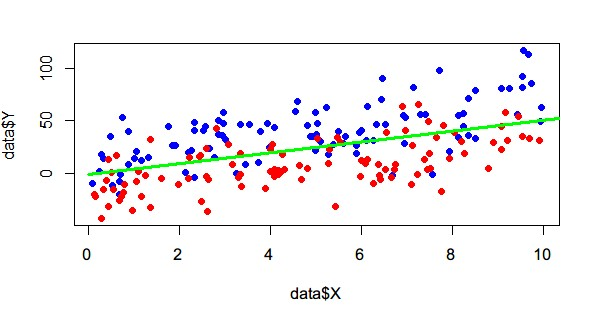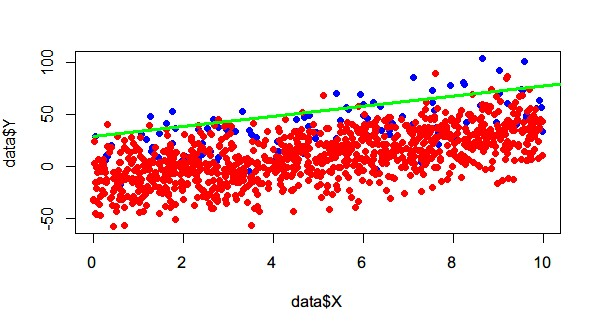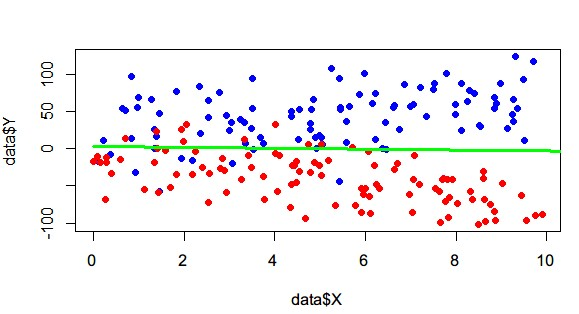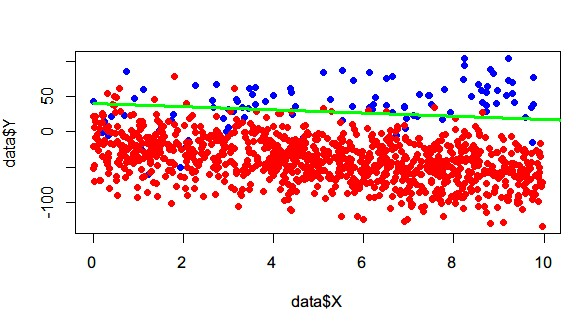LR模型常见问题小议

// 毕竟不是什么大牛，只是总结一下自己的一些认识和想法，如果有不正确的，还请大牛们斧正。

数据归一化

1. 归一化： (x-最小值)/(最大值-最小值)
2. 标准化： (x-平均数)/标准差

特征离散化&组合

离散化

A为1,0,0,0
B为0,1,0,0
C为0,0,1,0
D为0,0,0,1

0-10
10-20
20-30
……

120km/h匀速行驶时,油耗为7.81升/100km
90km/h匀速行驶时, 油耗为5.86升/100km
60km/h匀速行驶时, 油耗为4.12升/100km
30km/h匀速行驶时 ，油耗为4.10升/100km

小结

LR的劣势就是线性模型的线性假设过强，但我们发现通过上面这些trick，其实也可以学习”非线性“的特征，大大增强了LR的能力，所以LR才能这么流行。

正负样本不均衡问题

（注意这里只讨论LR，不要乱推广到其他算法）感觉怎么这问题有点像”玄学”了，坊间通用的说法是样本太不均衡是会有问题的，正负样本比例多大合适? 一些经验主义的说法是顶多负样本是正样本的几(5以内吧)倍。


LABEL_1_NUM <- 100
LABEL_0_NUM <- 100

K <- 5

x1 <-runif(LABEL_1_NUM, 0, 10)
x2 <-runif(LABEL_0_NUM , 0, 10)

D <-1
x2 <- rep(x2,D)
Y <- c( x1* K + rnorm(LABEL_1_NUM, mean=15, sd=19), x2 * K + rnorm(LABEL_0_NUM, mean=-15, sd=19) )
#Y <- c( x1* K + runif(LABEL_1_NUM, min=-15, max=50), x2 * K + runif(LABEL_0_NUM, min=-50, max=15) )

X <- c( x1, x2 )
label <- as.factor( c(rep(1,LABEL_1_NUM), rep(0,LABEL_0_NUM*D) ) )

data <- data.frame(X,Y, label)

model <- glm(label~Y+X, data=data, family='binomial', control = list(maxit = 600))

plot( data$X , data$Y , type="p", pch=19, col="red")
points( data$X[ data$label==1], data$Y[ data$label==1], type="p", pch=19, col="blue")
points( data$X[ data$label==0], data$Y[ data$label==0], type="p", pch=19, col="red")
co = coef(model)
lines(c(0,100),c(-co/co, -(co+co*100)/co), type="l", col='green', lwd=3)

print(co)
#截距
print(-co/co)
#斜率
print(-co/co)